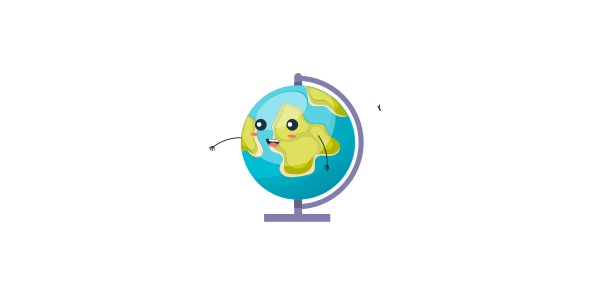# 5th Grade Computer Skills Test (2)

10 Questions | Total Attempts: 5174SettingsIt is important to have some knowledge on the use of computers as they are used all around us to make work easier. How conversant are you with the use of computers? Are you ready for the end of year test? Take up the quiz below and find out. Good luck!

• 1.
The intersection of a row and a column which can contain data within a spreadsheet is called:
• A.

Formula

• B.

Entry

• C.

Cross point

• D.

Cell

• 2.
A graph that can be used to compare the height of 15 sunflowers is a:
• A.

Bar graph

• B.

Data graph

• C.

Sorting graph

• D.

Comparing graph

• 3.
A multimedia presentation that moves in a straight line from image to image is called:
• A.

Non-linear

• B.

Linear

• C.

Correct imagery

• D.

Storyboard

• 4.
The horizontal divisions in a spreadsheet named with a number are called:
• A.

Cell

• B.

Sort

• C.

Entry

• D.

Row

• 5.
An application that is used to test "what if" questions is called a:
• A.

Database

• B.

• C.

Word processor

• D.

PowerPoint

• 6.
A graphic organizer that is used for planning and developing a multimedia report/presentation is called a:
• A.

Story web

• B.

Tree map

• C.

Storyboard

• D.

Linear map

• 7.
Telecommunication is the act of sending and receiving information such as:
• A.

Video

• B.

Sound

• C.

Pictures

• D.

All of the above

• 8.
The shape and style of text is called:
• A.

Font

• B.

View

• C.

Format

• D.

Graphic

• 9.
Organizing or sorting information in order from largest to smallest, Z to A or 9 to 1 is called:
• A.

Organization

• B.

Ascending

• C.

Size sorting

• D.

Descending

• 10.
An example of a formula in a spreadsheet is:
• A.

4 + 5 =

• B.

A6 + B2 =

• C.

= A6 : B2

• D.

= A6 + B2

Related TopicsBack to top